PHY294H - Lecture 38

The law of reflection can be understood by either the particle picture (think of an elastic collision of a particle with a hard surface) or in terms of waves (Use Huygen's principle). Note that at any interface, there is partial transmission, partial absorption and partial reflection. We shall not discuss absorption much. Optical materials are those which transmit most of the incident light, for these materials obsorption is considered a deleterious effect or a loss". Ideal mirrors have perfect reflection while perfect lenses have perfect transmission.

From the formula v = c/n, it is evident that light waves slow down inside materials. However if a source is emitting at frequency f, this frequency should be observed no matter where a observer is. Another way of stating this is that the number of wavefronts which pass an observer per unit time must be the same as the number of wavefronts produced by the source, per unit time. The frequency of light is thus the same inside a material as it is in air. If the frequency is constant, we have,(1)

This implies,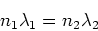(2)

This demonstrates that in high refractive index materials, the wavelength of light is shorter.

An extremely useful approach to geometrical optics is to use ray tracing. The ray associated with an EM wave is drawn perpendicular to the wavefronts for the wave. Almost everything that we shall do in geometrical optics is most easily understood using ray tracing. The key principle concerning rays in geometrical optics is Fermat's principle which states that: Light rays take the path which has the shortest transit time. Using this principle it is easy to prove the laws of reflection and refraction.

Total internal reflection

An important special case of Snell's law of refraction is that when light inside a material with refractive index n1 strikes an interface with refractive index n2<n1 at a sufficiently high angle, then reflection occurs instead of transmission. The condition for this "total internal reflection" is,(3)

This has solution,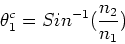(4)

The most extreme case is a diamond/air interface, where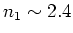and n2 = 1, in which case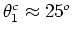. Any angle of incidence which is greater than this will internally reflect from the diamond/air interface. This means that light which enters diamond undergoes many internal reflections before it escapes. This is what gives diamond it appealing sparkle.

Another extremely important example is optical fibers. The simplest optical fibers consist of an inner region(core) of higher refractive index and an outer region(cladding) of lower refractive index. Light enters the fiber at high angles (in a cone of acceptance) and propagates along the fiber by internal reflection. Optical communications systems consist of: Solid state lasers which produces light from incoming electrical signals; A network of optical fibers which carries the signal to the target locations; Photodiodes which take incoming light and produce electrical signals from it. Optical fiber communications networks have many advantages over wireless communications.

It is important in some situations to screen out EM fields. This is achieved by placing a wire mesh around whatever needs to be protected. Actually any conducting surface will do provided the holes in the mesh are smaller than the wavelength of light which we wish to screen out.

Polarization

So far we have considered light which is linearly polarized. We have taken the electric field as being oscillatory in the x-direction. Now imagine placing a grid of straight conducting wires in the path of this wave. If the wires are oriented in the same direction as the electric field, a current is induced in the wire and the electric field is dissipated by these eddy currents. In quantum terminology the light is absorbed. However if the wires are oriented in the direction perpendicular to the electric field, there is little motion of the charges in the wire and hence the light can propagate through the wires. Polaroid glasses work in the same way. They are made of molecular wires, which are long conducting molecules oriented in one direction. The orientation of the molecules in polaroid glasses is determined by the properties of polarization under reflection, which we will come to a little later.

Malus's law

First consider a polarizer (i.e. the wires) to be at an angle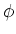to the direction of the linearly polarized light. We may then write, this is originally due to Malus,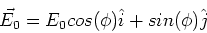(5)

The component which is along the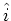direction is absorbed, while thecomponent is transmitted. The intensity of the tranmitted light is then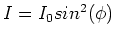. Let us now consider the arrangement in which Malus's law is usually states. Namely that we have two polarizers which are at an angle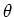to each other. The first polarizer transmits light which is polarized perpendicular to its conducting axis. If the second polarizer is at angle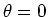to the first, then all of the light that comes through the first polarizer is transmitted. If the second is at 90o to the first, no light is transmitted. In terms of the relative angle between two polarizers, Malus's law is,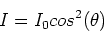(6)

Polarizers in series

An amusing feature of Malus's law, is that two polarizers at 90o to each other will block light, but if we place a third polarizer at 45o in between, light is transmitted, so that even though I2=0, we have,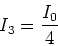(7)